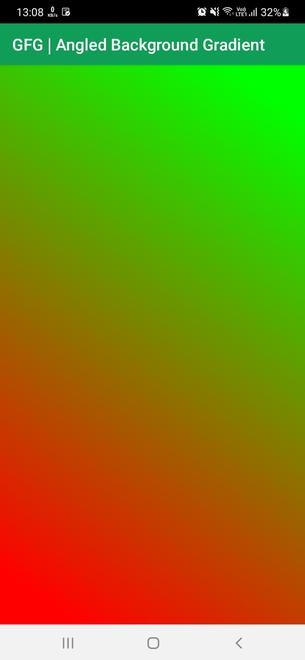# Angled Gradient Background in Android using Jetpack Compose

• Last Updated : 03 May, 2022

A Color Gradient is a color scheme that consists of two or more colors, where there is a color transition between any two colors. In Jetpack Compose, we can facilitate horizontal, vertical, and radial gradients with the help of in-built libraries. However, for anything out of these three, we need to design a custom program. A sample image is given below to get an idea about what we are going to do in this article.So in this article, we will show you how you could create an angled color gradient in Android using Jetpack Compose. Follow the below steps once the IDE is ready.

### Step by Step Implementation

Step 1: Create a New Project in Android Studio

To create a new project in Android Studio please refer to How to Create/Start a New Project in Android Studio. While choosing the template, select Empty Compose Activity. If you do not find this template, try upgrading the Android Studio to the latest version. We demonstrated the application in Kotlin, so make sure you select Kotlin as the primary language while creating a New Project.

Step 2: Working with the MainActivity.kt file

Go to the MainActivity.kt file and refer to the following code. Below is the code for the MainActivity.kt file. Comments are added inside the code to understand the code in more detail.

## Kotlin

 `package` `com.geeksforgeeks.angledgradient` `import` `android.os.Bundle``import` `androidx.activity.ComponentActivity``import` `androidx.activity.compose.setContent``import` `androidx.compose.foundation.layout.Arrangement``import` `androidx.compose.foundation.layout.Box``import` `androidx.compose.foundation.layout.Column``import` `androidx.compose.foundation.layout.fillMaxSize``import` `androidx.compose.material.*``import` `androidx.compose.ui.Alignment``import` `androidx.compose.ui.Modifier``import` `androidx.compose.ui.draw.drawBehind``import` `androidx.compose.ui.geometry.Offset``import` `androidx.compose.ui.graphics.Brush``import` `androidx.compose.ui.graphics.Color``import` `java.lang.Math.*``import` `kotlin.math.pow``import` `kotlin.math.sqrt` `class` `MainActivity : ComponentActivity() {``    ``override fun onCreate(savedInstanceState: Bundle?) {``        ``super``.onCreate(savedInstanceState)``        ``setContent {` `            ``// Creating a Simple Scaffold``            ``// Layout for the application``            ``Scaffold(` `                ``// Creating a Top Bar``                ``topBar = { TopAppBar(title = { Text(``"GFG | Angled Background Gradient"``, color = Color.White) }, backgroundColor = Color(``0xff0f9d58``)) },` `                ``// Creating Content``                ``content = {` `                    ``// Creating a Column Layout``                    ``Column(Modifier.fillMaxSize(), horizontalAlignment = Alignment.CenterHorizontally, verticalArrangement = Arrangement.Center) {` `                        ``// Create a Box Modifier and apply the gradient background function``                        ``// Colors are set to Red and Green and Angle is set to 45 Degrees clockwise``                        ``Box(Modifier.fillMaxSize().gradientBackground(listOf(Color.Red, Color.Green), angle = 45f))` `                    ``}``                ``}``            ``)``        ``}``    ``}` `    ``// Creating a customized function for angled color gradient``    ``private` `fun Modifier.gradientBackground(colors: List, angle: Float) = ``this``.then(``        ``Modifier.drawBehind {``          ` `            ``// Setting the angle in radians``            ``val angleRad = angle / 180f * PI``          ` `            ``// Fractional x``            ``val x = kotlin.math.cos(angleRad).toFloat()``            ` `            ``// Fractional y``            ``val y = kotlin.math.sin(angleRad).toFloat()` `            ``// Set the Radius and offset as shown below``            ``val radius = sqrt(size.width.pow(``2``) + size.height.pow(``2``)) / 2f``            ``val offset = center + Offset(x * radius, y * radius)` `            ``// Setting the exact offset``            ``val exactOffset = Offset(``                ``x = kotlin.math.min(offset.x.coerceAtLeast(0f), size.width),``                ``y = size.height - kotlin.math.min(offset.y.coerceAtLeast(0f), size.height)``            ``)` `            ``// Draw a rectangle with the above values``            ``drawRect(``                ``brush = Brush.linearGradient(``                    ``colors = colors,``                    ``start = Offset(size.width, size.height) - exactOffset,``                    ``end = exactOffset``                ``),``                ``size = size``            ``)``        ``}``    ``)``}`

Output:

You can see that we are able to create an angled color gradient angled at 45 Degrees.My Personal Notes arrow_drop_up